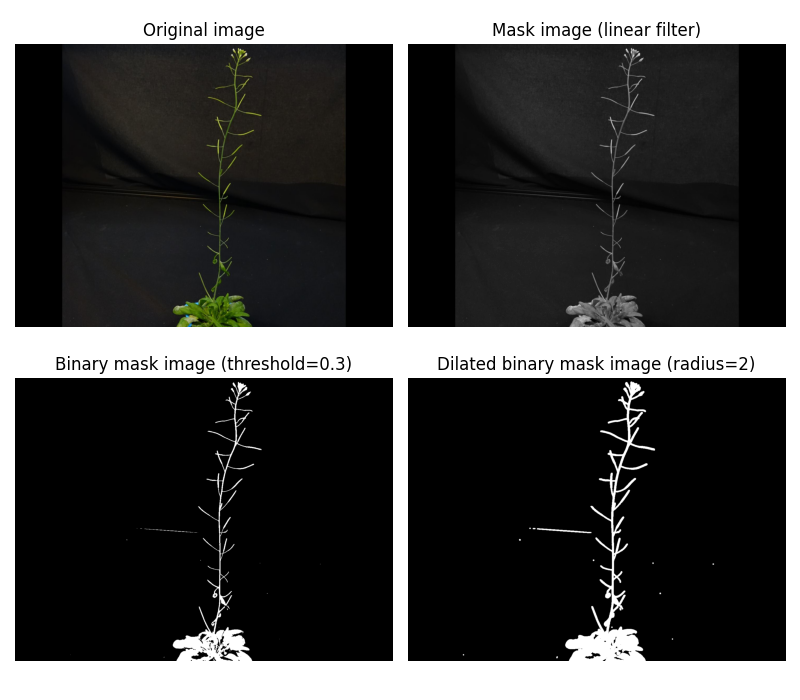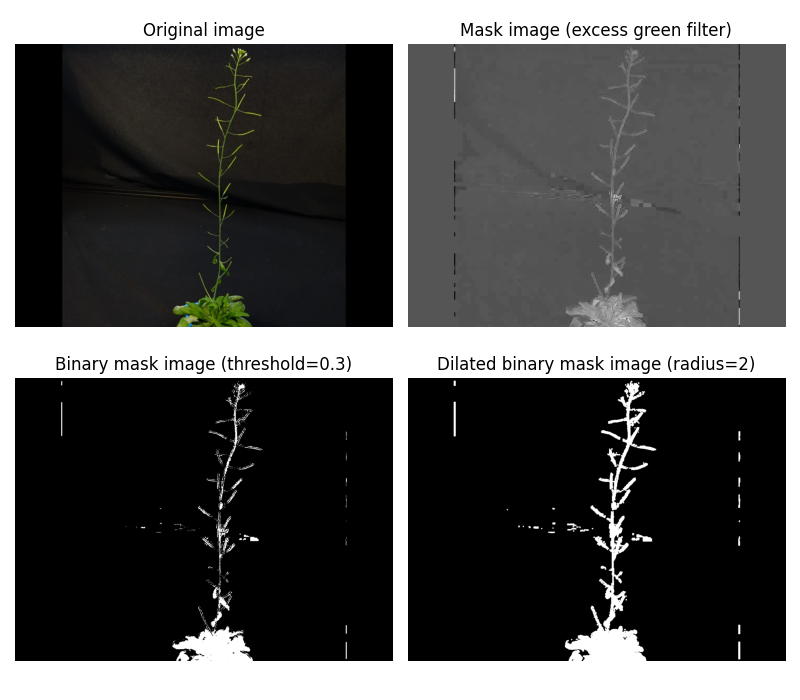Here we show the effects of the type of filter to use in the Mask task.

## Method linearLink

The equation for the linear filter method ($LF$) is as follows: $LF= c_r*R + c_g*G + c_b*B$, with $c_r$, $c_g$ & $c_b$ a coefficient in $[0., 1.]$ to apply to the corresponding channel.

Then we apply a high-pass threshold to binarize the image and create a mask locating the plant in the image. Finally, we use a binary dilation to enlarge the masked area.

Here is an example of what the linear filter does when used in combination with a binarization step and a dilation step, as in the Masks task:The source code for this figure is as follows:

import matplotlib.pyplot as plt
from plant3dvision import test_db_path
from plant3dvision.proc2d import linear, dilation
path = test_db_path()
rgb_coefs = [0.1, 1., 0.1]
filter_img = linear(img, rgb_coefs)  # apply linear filter
threshold = 0.3
mask = filter_img > threshold  # convert to binary mask using threshold
fig, axes = plt.subplots(2, 2, figsize=(8, 7))
axes[0, 0].imshow(img)
axes[0, 0].set_title("Original image")
axes[0, 1].imshow(filter_img, cmap='gray')
[ax.set_axis_off() for ax in axes.flatten()]
plt.tight_layout()


Important

Pay attention to the values used for rgb_coefs, threshold and dilation.

## Method excess_greenLink

This filter was published in: Woebbecke, D. M., Meyer, G. E., Von Bargen, K., & Mortensen, D. A. (1995). Color indices for weed identification under various soil, residue, and lighting conditions. Transactions of the ASAE, 38(1), 259-269.

The equation for the excess green method ($EG$) is as follows: $EG= 2*g-r-b$, with:

• $r = R/(R+G+B)$
• $g = G/(R+G+B)$
• $b = B/(R+G+B)$
• Then we apply a high-pass threshold to binarize the image and create a mask locating the plant in the image. Finally, we use a binary dilation to enlarge the masked area.

Here is an example of what the excess_green filter does when used in combination with a binarization step and a dilation step, as in the Masks task:The source code for this figure is as follows:

import matplotlib.pyplot as plt
from plant3dvision import test_db_path
from plant3dvision.proc2d import excess_green, dilation
path = test_db_path()
filter_img = excess_green(img)  # apply excess_green filter
threshold = 0.3
mask = filter_img > threshold  # convert to binary mask using threshold
fig, axes = plt.subplots(2, 2, figsize=(8, 7))
axes[0, 0].imshow(img)
axes[0, 0].set_title("Original image")
axes[0, 1].imshow(filter_img, cmap='gray')
axes[0, 1].set_title("Mask image (excess green filter)")

Pay attention to the values used for threshold and dilation.